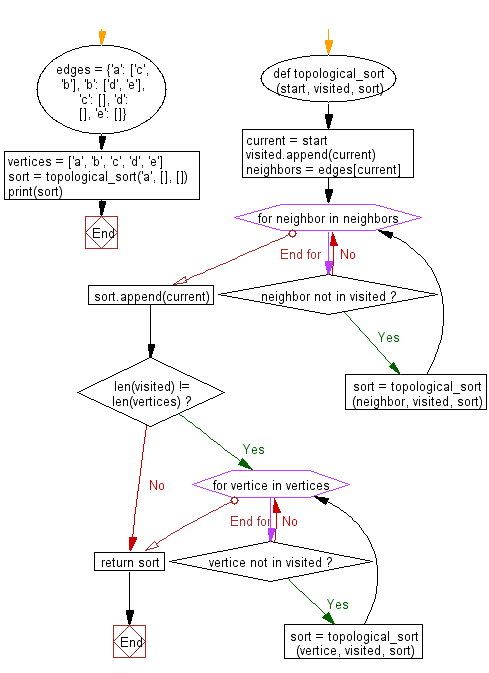﻿ Python: Sort a list of elements using Topological sort - w3resource# Python: Sort a list of elements using Topological sort

## Python Search and Sorting : Exercise-22 with Solution

Write a Python program to sort a list of elements using Topological sort.

Sample Solution:

Python Code:

``````# License https://bit.ly/2InTS3W
#     a
#    / \
#   b  c
#  / \
# d  e
edges = {'a': ['c', 'b'], 'b': ['d', 'e'], 'c': [], 'd': [], 'e': []}
vertices = ['a', 'b', 'c', 'd', 'e']
def topological_sort(start, visited, sort):
"""Perform topolical sort on a directed acyclic graph."""
current = start
visited.append(current)
neighbors = edges[current]
for neighbor in neighbors:
# if neighbor not in visited, visit
if neighbor not in visited:
sort = topological_sort(neighbor, visited, sort)
# if all neighbors visited add current to sort
sort.append(current)
# if all vertices haven't been visited select a new one to visit
if len(visited) != len(vertices):
for vertice in vertices:
if vertice not in visited:
sort = topological_sort(vertice, visited, sort)
# return sort
return sort

sort = topological_sort('a', [], [])
print(sort)
```
```

Sample Output:

```['c', 'd', 'e', 'b', 'a']
```

Flowchart:Python Code Editor:

What is the difficulty level of this exercise?

Test your Programming skills with w3resource's quiz.

﻿

## Python: Tips of the Day

The Zip() Function:

```>>> students = ('John', 'Mary', 'Mike')
>>> ages = (15, 17, 16)
>>> scores = (90, 88, 82, 17, 14)
>>> for student, age, score in zip(students, ages, scores):
...     print(f'{student}, age: {age}, score: {score}')
...
John, age: 15, score: 90
Mary, age: 17, score: 88
Mike, age: 16, score: 82
>>> zipped = zip(students, ages, scores)
>>> a, b, c = zip(*zipped)
>>> print(b)
(15, 17, 16)
```